# Basic Rotational QuantitiesIn addition to any tangential acceleration, there is always the centripetal acceleration: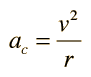The angular displacement is defined by: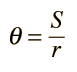For a circular path it follows that the angular velocity is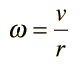and the angular acceleration is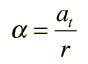where the acceleration here is the tangential acceleration.
The standard angle of a directed quantity is taken to be counterclockwise from the positive x axis.
Index

Centripetal acceleration

 HyperPhysics***** Mechanics ***** Rotation R Nave
Go Back

# Angular Velocity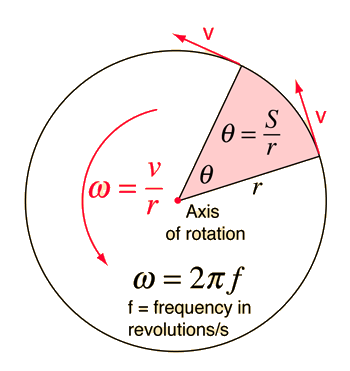Angular velocity can be considered to be a vector quantity, with direction along the axis of rotation in the right-hand rule sense.

### Vector angular velocity

For an object rotating about an axis, every point on the object has the same angular velocity. The tangential velocity of any point is proportional to its distance from the axis of rotation. Angular velocity has the units rad/s.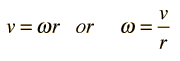Angular velocity is the rate of change of angular displacement and can be described by the relationship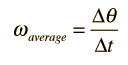and if v is constant, the angle can be calculated from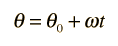Index

Basic rotational quantities

 HyperPhysics***** Mechanics ***** Rotation R Nave
Go Back

# Description of Rotation

Rotation is described in terms of angular displacement, time, angular velocity, and angular acceleration. Angular velocity is the rate of change of angular displacement and angular acceleration is the rate of change of angular velocity. The averages of velocity and acceleration are defined by the relationships:

 Average angular velocity: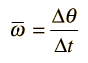Average angular acceleration: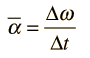where the Greek letter delta indicates the change in the quantity following it.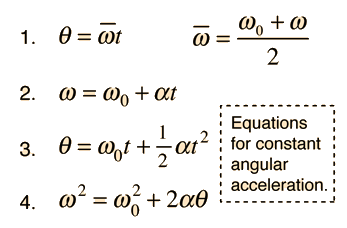A bar above any quantity indicates the average value of that quantity. If α is constant, equations 1, 2, and 3 represent a complete description of the rotation. Equation 4 is obtained by a combination of the others.

You might want to try a numerical exploration of these equations and see them stated in words.

Index

 HyperPhysics***** Mechanics ***** Rotation R Nave
Go Back

# Rotation Equations

These rotation equations apply only in the case of constant angular acceleration. It is assumed that the angle is zero at t=0 and that the motion is being examined at time t.

angular displacement*θ = average angular velocity x time* t

angular velocity ω = initial angular velocity* + ang. acceleration α x time

ang. displacement = initial ang. velocity x t + 1/2 ang. accel. x time2

*These quantities are assumed to be given unless they are specifically clicked on for calculation.

You can probably do all this calculation more quickly with your calculator, but you might find it amusing to click around and see the relationships between the rotational quantities. It's like a game to see if you can set up a consistent set of parameters. Associated with each active text is a Javascript calculation routine.

Index

 HyperPhysics***** Mechanics ***** Rotation R Nave
Go Back